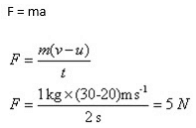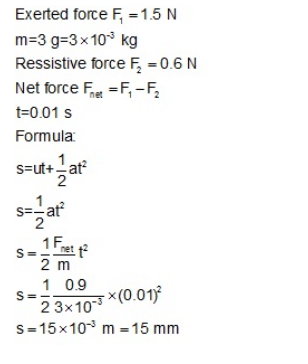Test: Newton's Second Law Of Motion

# Test: Newton's Second Law Of Motion - Class 9

Test Description

## 20 Questions MCQ Test Science Class 9 - Test: Newton's Second Law Of Motion

Test: Newton's Second Law Of Motion for Class 9 2023 is part of Science Class 9 preparation. The Test: Newton's Second Law Of Motion questions and answers have been prepared according to the Class 9 exam syllabus.The Test: Newton's Second Law Of Motion MCQs are made for Class 9 2023 Exam. Find important definitions, questions, notes, meanings, examples, exercises, MCQs and online tests for Test: Newton's Second Law Of Motion below.
Solutions of Test: Newton's Second Law Of Motion questions in English are available as part of our Science Class 9 for Class 9 & Test: Newton's Second Law Of Motion solutions in Hindi for Science Class 9 course. Download more important topics, notes, lectures and mock test series for Class 9 Exam by signing up for free. Attempt Test: Newton's Second Law Of Motion | 20 questions in 20 minutes | Mock test for Class 9 preparation | Free important questions MCQ to study Science Class 9 for Class 9 Exam | Download free PDF with solutions
 1 Crore+ students have signed up on EduRev. Have you?
Test: Newton's Second Law Of Motion - Question 1

### The S.I. unit of force is

Detailed Solution for Test: Newton's Second Law Of Motion - Question 1

Force is the product of the ass and acceleration.
Therefore, Force = Mass x Acceleration
The SI unit of Force is  kg.m/s² or Newton
1 Newton =  10⁵ Dyne

Test: Newton's Second Law Of Motion - Question 2

### Two balls A and B, of masses m and 2m are in motion with velocities 2v and v respectively. Compare the force needed to stop them in the same time

Detailed Solution for Test: Newton's Second Law Of Motion - Question 2
The acceleration produced in a body is directly proportional to the force acting on it and inversely proportional to the mass of the body. If the mass of the body is doubled, its acceleration will be halved. And if the mass is halved then acceleration will get doubled. So the provided force remains same.
Test: Newton's Second Law Of Motion - Question 3

### Find the time taken by a body of mass 16 kg to come to rest from a uniform velocity of magnitude 10 m/s, when a force of 4N is applied continously

Detailed Solution for Test: Newton's Second Law Of Motion - Question 3

F =  4 N,  u = 10 m / s  ,  m = 16 kg
v= u + at
0 = 10 + (-1 /4) t
0=  10- 1 /4 t
t  =   40 s

Test: Newton's Second Law Of Motion - Question 4

If the force acting on the body is zero. Its momentum is:

Detailed Solution for Test: Newton's Second Law Of Motion - Question 4

The relationship also implies the conservative of momentum : when the net force on the body is zero, the momentum of the body is constant. Any net force is equal to the rate of change of the momentum.

Test: Newton's Second Law Of Motion - Question 5

Which of the following has the largest momentum?

Detailed Solution for Test: Newton's Second Law Of Motion - Question 5
Because the momentum is the force created by the mass and velocity of a body for example if a table tennis ball hit you it will not hurt because it have lesser mass and lesser velocity and if a cricket ball hits you it will hurt because the cricket ball have more mass and velocity. so by this we can say that the pickup truck travelling down the highway will have greater momentum as it have more mass than any other and also it have more velocity than others as it is in higher speed. I hope you learned something
Test: Newton's Second Law Of Motion - Question 6

What force can change the velocity of a body of mass 1kg from 20 m/s to 30m/s in 2 seconds?

Detailed Solution for Test: Newton's Second Law Of Motion - Question 6Test: Newton's Second Law Of Motion - Question 7

Find the time for which a force of 1kgwt acts on a body of mass 1 kg moving with a uniform speed of 4m/s to stop the body.

Detailed Solution for Test: Newton's Second Law Of Motion - Question 7
Stopping force is F = – 10 N.

Here, F = m[(v - u)/t]
t = 1 kg [(0 - 4m/s)/-10N]
= 1 kg [0.4 m/s]
So, t = 0.4 sec
Test: Newton's Second Law Of Motion - Question 8

A resistive force of 16 N acts on a ball of mass 40 g continuously. If the initial velocity of the ball is 24 ms-1 ,the time taken by it to come to rest is:

Detailed Solution for Test: Newton's Second Law Of Motion - Question 8
Mass of ball: m= 40 g = 0.040kg
resistive force: F = -16 N
thus resistive acceleration, a = F/m (because force, F= ma)
thus a = -400m/s2
initial velocity, u = 24m/s
final velocity, v = 0m/s
Thus according to first equation of motion, v = u+at (t=timetaken)
t= 0.06s
Test: Newton's Second Law Of Motion - Question 9

The acceleration of an object is inversely proportional to:

Detailed Solution for Test: Newton's Second Law Of Motion - Question 9

For the same force, an object with twice the mass will have half the acceleration. If it had three times the mass, the same force will produce one-third the acceleration. Four times the mass gives one-fourth of the acceleration, and so on.
This type of relationship between quantities (double one, get half the other) is called an inverse proportion or inverse variation. In other words, then:
The acceleration is inversely proportional to the mass of the object.
Newton's Second Law

"If the net force on an object is not zero, the object will accelerate. The direction of the acceleration is the same as the direction of the net force. The magnitude of the acceleration is directly proportional to the net force applied, and inversely proportional to the mass of the object."

Test: Newton's Second Law Of Motion - Question 10

Quantitative expression of force is given by:

Detailed Solution for Test: Newton's Second Law Of Motion - Question 10

Force can be calculated from Newton’s second law of motion which gives that force is the product of mass and acceleration.

Test: Newton's Second Law Of Motion - Question 11

If the same force acts on two bodies of different masses which are initially at rest and gain same displacement, then the ratio of time for which these forces act is:

Test: Newton's Second Law Of Motion - Question 12

A man throws a ball weighing 200 g vertically upwards with a speed of 10m/s. Its momentum at the highest point of its flight will be:

Detailed Solution for Test: Newton's Second Law Of Motion - Question 12
As on highest point velocity is zero .Hence momentum will also be zero as it is the product of mass and velocity of a body.
Test: Newton's Second Law Of Motion - Question 13

In high jump competition the athlete is made to fall on a cushioned bed to:

Detailed Solution for Test: Newton's Second Law Of Motion - Question 13

In a high jump athletic event, the athletes are made to fall either on a cushioned bed or sand bed. This is to increase the time that the athletes fall to stop after making the jump. This decreases the rate of change of momentum and hence the force. As a result, the athlete does not get injured.

Test: Newton's Second Law Of Motion - Question 14

Which of the following is the relation between SI unit of gravitational force and Newton?​

Detailed Solution for Test: Newton's Second Law Of Motion - Question 14
In SI system, the gravitational unit of force is kilogram – weight. It is that force which when acting on a body of mass 1 kg produces an acceleration of 9.8 m s^-2
Test: Newton's Second Law Of Motion - Question 15

A carpenter exerts a force of magnitude 1.5 N at right angles to the surface of a nail of mass 3 gram to drive it into the wood. If wood offers a resistive force of 0.6 N and the time of interaction of hammer and the nail 0.01 s, the depth through which the nail penetrates is

Detailed Solution for Test: Newton's Second Law Of Motion - Question 15Test: Newton's Second Law Of Motion - Question 16

Change in momentum when a car weighing 700kg changes its speed from 100m/s to 200 m/s is:

Detailed Solution for Test: Newton's Second Law Of Motion - Question 16

Change in momentum = mv - mu
When m = 7000kg, v= 200m/s, u = 100 m/s
Now, 700 * 200 - 700 x 100 = 140000 - 70000 = 70000 kg. m/s

Test: Newton's Second Law Of Motion - Question 17

Momentum of a body of mass 0.5 kg moving with a speed of 10 m/s is​

Detailed Solution for Test: Newton's Second Law Of Motion - Question 17

We know , p=mv Here m=0.5 kg & v=10 m/s . So p=0.5*10=5 Kg m/s

Test: Newton's Second Law Of Motion - Question 18

The relation between acceleration, mass and force is given by:

Detailed Solution for Test: Newton's Second Law Of Motion - Question 18
The acceleration is directly proportional to f and inversely proportional to m and so the force applied on a mass of a body let to movement that's why here a is directly proportional to f
Test: Newton's Second Law Of Motion - Question 19

What is the momentum of a body of mass 2m and velocity v/2?

Detailed Solution for Test: Newton's Second Law Of Motion - Question 19

We know; P = mv
If m = 2 m ;   v= v/2
P  =  (2m) (v/2)
P = mv

Test: Newton's Second Law Of Motion - Question 20

An object of mass 20 kg is moving with a velocity of 10 m/s. Its momentum will be:

Detailed Solution for Test: Newton's Second Law Of Motion - Question 20
Momentum p = mass x velocity
Given mass m=20 kg and velocity v = 10 m/s

So,
p = 20 kg x 10 m/s = 200 kg.m/s

## Science Class 9

66 videos|355 docs|97 tests
Information about Test: Newton's Second Law Of Motion Page
In this test you can find the Exam questions for Test: Newton's Second Law Of Motion solved & explained in the simplest way possible. Besides giving Questions and answers for Test: Newton's Second Law Of Motion, EduRev gives you an ample number of Online tests for practice

## Science Class 9

66 videos|355 docs|97 tests